# Shiny

## Our Collection of Apps

 Regression Correlation and Regression Game Multiple Regression Visualization Inference Benford's Law: Data Examples (Census and Stock Exchange) Benford's Law: Sequences Hot Hand Phenomenon: Randomization-based Analysis Length/Coverage Optimal Confidence Intervals Performance of the Wilcoxon-Mann-Whitney Test vs. t-test t-test with diagnostics Testing Violation of the Constant Variance Condition for ANOVA Probability and Randomness Chaos Game: Two Dimensions Chaos Game: Three Dimensions Gambler's Ruin Longest Run of Heads or Tails Distribution Theory and Estimation Maximum Likelihood Estimation for the Binomial Distribution Probability Distribution Viewer Random Variable Generation Sampling Distributions of Various Statistics Special Topics Heaped Distribution Estimation Hierarchical Models Population Genetics
Reference: Doi, J., Potter, G., Wong, J., Alcaraz, I., and Chi, P. (2016) “Web Application Teaching Tools for Statistics Using R and Shiny.” Technology Innovations in Statistics Education 9(1). Available at http://escholarship.org/uc/item/00d4q8cp. Corresponding Author: Jimmy Doi

## Regression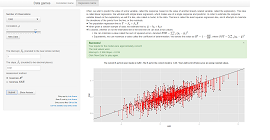Correlation and Regression Game

Correlation and regression are two closely related topics. Often they are taught to students at about the same time, thus giving them the opportunity to explore both topics in the same application is an intuitive choice. The goals of this application are then two. The first is to give students with an experience where they can easily visualize different graphical representations of the full range of correlation values. The second is to give students an interactive experience where they can learn to recognize and apply the different features that determine the solutions to both correlation and regression problems.

The app provides both topics separated by different tabs. In the correlation portion of the app, a random set of data, with a certain correlation, is generated and user must attempt to correctly guess the correlation of the data. The user can mouse over the individual data points if they wish to manually calculate the correlation. Once the user submits an answer they are notified whether they guess correctly on not through a popup alert, this feature appears in both portions of the application. In the regression portion of the app, again random data is generated without a superimposed regression line. The user must input values for the slope and intercept, after which a line and residuals are drawn. After the user has already submitted an estimate once, they may edit the parameter estimates interactively to see how the changes effect the residuals. The app then notifies the user whether they were correct or not in guessing the least squares regression line.Multiple Regression Visualization

Scatterplots are often useful to visualize the relationship between two quantitative variables. However, with Multiple Regression, there are more than one predictor variables used to model one response variable. Thus, a simple scatterplot is no longer adequate to graphically represent all of the variables. In the case of two predictor variables, we can illustrate this in three dimensions, or also in two dimensions with appropriate color schemes. This applet shows us these illustrations for a variety of datasets.

## Inference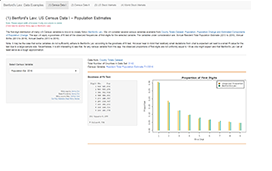Benford's Law: Data Examples (Census I, Census II, US and World Stock Exchange)

Census I: The first-digit distribution of many US Census variables is known to closely follow Benford's Law. We will consider several census variables available from County Totals Dataset: Population, Population Change and Estimated Components of Population Change. The app will apply a goodness of fit test of the observed frequencies of first-digits for the selected variable. The variables under consideration are: Annual Resident Total Population Estimate (2013 to 2016), Annual Births (2013 to 2016), Annual Deaths (2013 to 2016).

Census II: We also consider several census variables available from US Census State & County QuickFacts. The app will apply a goodness of fit test of the observed frequencies of first-digits for the selected variable. The variables under consideration are: Housing Units (2013), Households (2008-12), Veterans (2008-12), Nonemployer Establishments (2012), Private Nonfarm Establishments (2012), Private Nonfarm Employment (2012), Retail Sales (2007).

US Stock Markets: For an analysis of US stock market data the app will download information from the Wall Street Journal website from the most recent end of day market data. The data will be based on various market variables for all companies listed in one of four stock markets. The app will apply a goodness of fit test of the observed frequencies of first-digits for the selected variable in the specified stock market.

World Stock Markets: A similar goodness of fit analysis is done for market variables from various world stock markets based on data accessed from investing.com. For the selected stock market, if trading is active at the point of data access, the results will be based on the most current market data. If the market is closed at point of access, then all information will be based on the most recent end of day market data.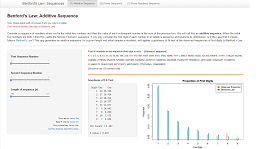Benford's Law: Sequences (Additive, Power, Prime Number)

This app examines the first-digit distribution of various sequences: Additive, Power, and Prime Number

Additive Sequence: Consider a sequence of numbers where we fix the initial two numbers and then the value of each subsequent number is the sum of the previous two. We will call this an additive sequence. When the initial two numbers are both 1 then this yields the famous Fibonacci sequence. If you only consider the first digit of each number in an additive sequence and examine its distribution, is it the case that it closely follows Benford's Law? This app generates an additive sequence, for a given length and initial sequence numbers, and applies a goodness of fit test of the observed frequencies of first digits to Benford's Law.

Power Sequence: Consider a sequence of the form b1, b2, …, bn, where b is called the base. We will call this a power sequence. If you only consider the first digit of each number in a power sequence and examine its distribution, is it the case that it closely follows Benford's Law? This app generates the power sequence, for a given b and n, and applies a goodness of fit test of the observed frequencies of first digits to Benford's Law.

Prime Number Sequence: Consider the sequence of prime numbers less than or equal to some power of 10. An article from 2009 shows that the distribution of the first digit of these prime numbers is well described by what's known as Generalized Benford's Law (GBL) . This app generates the prime numbers less than or equal to 103, 104, 105, or 106 and applies a goodness of fit test of the observed frequencies of first digits to GBL.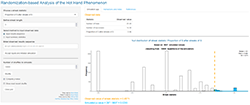Hot Hand Phenomenon: Randomization-based Analysis

Many basketball players and fans alike believe in the "hot hand" phenomemon: the idea that making several shots in a row increases a player's chance of making the next shot. Does the hot hand in basketball really exist? This app can be used to perform a statistical test for "hot hand" type behavior in sequences of success/failure trials, such as the shot attempts of a basketball player. A data set containing the results of each shot attempt for players in the NBA Three Point Contest from 2013 through 2017 is included.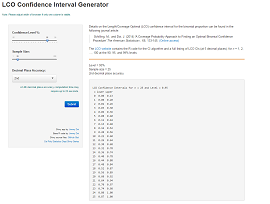Length/Coverage Optimal (LCO) Confidence Intervals

In 2014, Schilling and Doi developed a binomial confidence interval procedure that produces coverage probabilities always at least equal to the stated confidence level (e.g., a strict method), and which, among all procedures that have this property, give confidence intervals having the minimum possible average length and the highest possible coverages. They called this the LCO method (for length/coverage optimal). This Shiny app generates LCO confidence intervals for any n = 1, 2, ..., 200 and any confidence level between 80% and 99%. The user may select the accuracy of the intervals to be at the 2nd, 3rd, or 4th decimal place.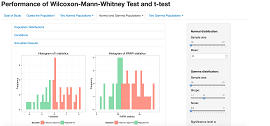Performance of Wilcoxon-Mann-Whitney Test vs. t-test

The goal of this app is to compare the performance of a nonparametric to a parametric test for the difference in two population means. Specifically, performance is measured in the app either by Type I error rate or power, and the two respective tests for comparison are the Wilcoxon-Mann-Whitney (WMW) test and the two-sample t-test. Recall that for the test conditions to be satisfied, the two-sample t-test requires either the two population distributions to be normal or large enough sample sizes while the WMW test requires the two population distributions to have the same shape. Users have the option to produce different scenarios and conclude the better test either through a lower Type I error rate (if the two population means are the same) or a higher power (if they are not).

When users first launch the app, they are presented with the goal of the study. Then, a game demonstrates to users the difficulty of identifying the population distributions of sample data. Following the first two introductory tabs, users can proceed to comparing performance. They have the option to choose a tab corresponding to their choice of the population distributions. Within each tab, either a single comparison or comparisons over a range can be conducted. The settings available for users to adjust are sample sizes, population means, significance level, number of simulations, and range of comparison values. In addition, visualizations are implemented to communicate results to users. For a single comparison, the outputs are distributions of the test statistics and gauges. For comparisons over a range, the output illustrates the performance of the two tests in each comparison.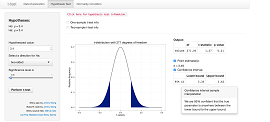t-test with diagnostics

This app focuses on conducting a t-test and checking the normality condition. Both the one-sample and two-sample t-tests are implemented in this app. Recall that for the t-test to be valid either sample size(s) need to be large enough or the population distribution(s) needs to be a Normal distribution. To begin the app, data configuration is required. Users have the choice to either use sample data or upload their own data when first launching the app. Customization is needed in respect to the uploaded data. After selecting their option, users can proceed to visualizing the data. A histogram is presented for one sample while comparative boxplots are presented for two samples. In addition, summary statistics are also available for display.

The hypothesis test tab displays the null and alternative hypotheses. The settings available for users to adjust are the hypothesized value, the direction for the alternative hypothesis, and the significance level. For users who are not familiar with the concept of the hypothesis test, they can click on a link that shows information in a popover. Additional information on the one-sample and two-sample t-tests is also available. When users have run the t-test, the output includes items such as the shaded t-distribution, t-statistic, and the p-value. The point estimate(s) and confidence interval can also be outputted by users’ request. In the normality condition tab, the Shapiro-Wilk normality test is performed and a Q-Q plot is displayed. In all relevant outputs throughout the app, sample interpretations from popovers are included for users to understand the results of the hypothesis test.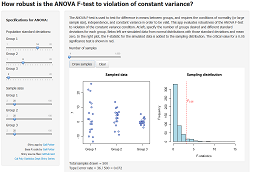Testing Violation of the Constant Variance Condition for ANOVA

The ANOVA F-test is used to test for difference in means between groups, and requires the conditions of normality (or large sample size), independence, and constant variance.  A common rule of thumb for the constant variance condition is that the ratio of largest to smallest standard deviation is less than or equal to two.  This application implements a user-guided simulation study to assess the consequences of non-constant variance on the Type I error rate of the ANOVA F-test.  The application enables the user to visualize data with different standard deviations, reinforces the concepts of sampling distribution, null distribution, and Type I error, and allows the user to uncover a rule of thumb for the constant variance condition.

At left, the user specifies standard deviations for three hypothetical populations and sample sizes to be drawn from each of the populations.  When the user presses the “Draw samples” button, data will be simulated from normal distributions with mean zero and the specified standard deviations and sample sizes and displayed in dot plots in the left graph.  The ANOVA F-statistic for the simulated data is plotted in the graph at right, and the critical value for a 0.05-level hypothesis test is shown in red.  As more samples are drawn (with the option to draw up to 1,000 samples at a time), more F-statistics are plotted in the sampling distribution on the right.  The Type I error rate is estimated as the proportion of samples for which the null hypothesis was rejected, and is displayed below the graphs.  Below the graphs (not included in the picture above) is guidance for a suggested series of simulation studies allowing the user to compare different specifications systematically and uncover the rule of thumb for the constant variance condition.

## Probability and Randomness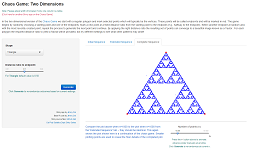Chaos Game: Two Dimensions

In the two dimensional version of the Chaos Game we start with a regular polygon and mark selected points which will typically be the vertices. These points will be called endpoints and will be marked in red. The game begins by randomly choosing a starting point and one of the endpoints. Mark a new point at a fixed distance ratio from the starting point to the endpoint (e.g., halfway to the endpoint). Select another endpoint at random and, with the most recently created point, repeat the process to generate the next point and continue. By applying the right distance ratio the resulting set of points can converge to a beautiful image known as a fractal. For each polygon the required distance ratio to yield a fractal will be provided, but try different settings to see what other patterns may arise!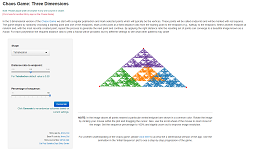Chaos Game: Three Dimensions

In the three dimensional version of the Chaos Game we start with a regular polyhedron and mark selected points which will typically be the vertices. These points will be called endpoints and will be marked with red squares. The game begins by randomly choosing a starting point and one of the endpoints. Mark a new point at a fixed distance ratio from the starting point to the endpoint (e.g., halfway to the endpoint). Select another endpoint at random and, with the most recently created point, repeat the process to generate the next point and continue. By applying the right distance ratio the resulting set of points can converge to a beautiful image known as a fractal. For each polyhedron the required distance ratio to yield a fractal will be provided, but try different settings to see what other patterns may arise!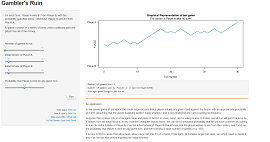Gambler's Ruin

The Gambler’s Ruin is a well-known problem that can be used to illustrate a variety of probability concepts.

Two players are playing a game against each other, betting the same amount on each turn (here, we use \$1). On each turn of the game, Player A has a fixed probability p of winning \$1 from Player B, where 0<p<1. The probability that Player B will win \$1 from Player A is 1-p. Player A and Player B each start with some initial fortune (which may or may not be equal to each other), and the game continues until one player has all of the money.

The Gambler’s Ruin problem is useful for teaching conditional probability, Markov chains, and for simply visualizing a stochastic process. This app shows a graphical representation of one iteration of the Gambler’s Ruin, and also can simulate many runs under a variety of settings that may be manipulated, to obtain simulated estimates of the average length of a game, and the probability that Player A will win under those settings. In a mathematical statistics class, the simulated estimates from this app could be used to corroborate analytic solutions.Longest Run of Heads or Tails

One popular class activity to help students understand chance behavior is to observe the runs of consecutive heads or tails in a sequence of coin flips. When asked to write down a simulated sequence of 100 tosses of a fair coin, most students are hesitant to create runs of heads or tails exceeding 4. Students are often surprised to find that the longest run of heads or tails turns out to be much higher based on 100 tosses of an actual coin.

This Shiny app allows the user to simulate the outcomes of a fair coin flipped n times (n = 10, 20, ..., 400). In an accompanying plot of outcomes any runs of at least a specified length are marked in color, and the length of the longest run is displayed. The user can easily re-randomize the sequence of coin flips and quickly get a sense of typical longest run values. From the plot students may also be quite surprised to see how many long runs occur in the sequence.

The user may choose to display the predicted approximate length of the longest run and an approximate 95% prediction interval for the length of the longest run. Details on these two estimators can be found in Schilling (1990). See Schilling (2012) for a more recent and related article.

## Distribution Theory and Estimation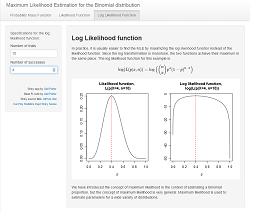Maximum Likelihood Estimation for the Binomial Distribution

This app provides an introduction to the concept of maximum likelihood estimation by working through the example of the binomial distribution. The first tab shows the probability mass function (pmf) of the binomial distribution. The user specifies the parameters to see various pmfs, and is guided to understand that this function takes the number of successes (x) as input and provides a probability as output.

The pmf is then contrasted with the likelihood function in the second tab. Here the user specifies the fixed "parameters" (x and n) for the likelihood function, and the likelihood curve is graphed. Here the user sees that the input to the function is p rather than x, and the text explains that inputs and parameters have effectively been switched. The user is guided to input various values of x and discover that the likelihood function is always maximized at p=x/n. The third tab displays the likelihood and log likelihood side-by-side so the user understands they achieve the maximum in the same location.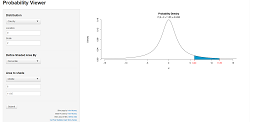Probability Distribution Viewer

Probability distributions, p-values, and percentiles are fundamental topics taught to introductory statistics students. Often, students are presented these topics with static images in textbooks, but frequently do not have access to a dynamic and interactive tool they can use for exploration. For example, in the case of p-values, introductory students are frequently shown how to use tables to obtain a range of possible p-values associated with their test statistic along with a guiding image, but this is often difficult for students to understand. The goal of this application is to provide the student with an intuitive, simple, and comprehensive visualization of the three aforementioned topics. At the moment, many but not all continuous distributions (Beta, Cauchy, Chi-Squared, Exponential, F, Gamma, Logistic, Log Normal, Normal, Student’s t, Uniform, and Weibull) are available in the application. Support for discrete may be added in the future.

When the app first renders, the user is shown by default the standard normal distribution. The student may vary the both the distribution and parameters corresponding to the distribution of their choice from the options at the top under "Distribution." This enables the student to see how the shape of their selected distribution changes as these values change. For students that are interested in visualizing probabilities and percentiles, the probability viewer app easily allows the student to select between two types of inputs. The student can also select between different shaded tail visualizations for the inputted percentiles or probabilities. Whether the student chooses to input a percentile or probability, the app will automatically calculate the value that the student did not input, corresponding to the one was. After inputting all required values, a graph appears with the appropriate distribution, the percentile and probability pairs, and the appropriate shading.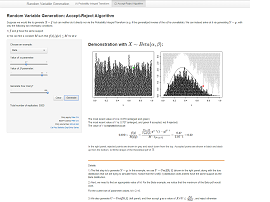Random Variable Generation

The Probability Integral Transform and the Accept-Reject Algorithm are two methods for generating a random variable with some desired distribution. This Shiny app demonstrates how they work, through two examples of each method.

For the Accept-Reject Algorithm (shown above), the examples demonstrated in this app are the Beta distribution and the truncated Normal distribution. A side-by-side plot shows each point that has been generated. Users have the option to generate one replicate at a time, to examine and understand the mechanics of how the algorithm is accomplishing its task, with details of each replicate given below the plots. Additionally, up to 500 replicates can be generated at once, to build towards a greater representation of points and confirm that the algorithm does in fact result in the desired distribution.

The Probability Integral Transform (not shown) is demonstrated with the Exponential distribution, and an arbitrary, unnamed distribution. In this demonstration, users again have the option to generate one replicate at a time, with side-by-side plots showing each point, and details of each replicate given below the plots. Users can also generate up to 500 replicates at once to view the overall distribution that is produced.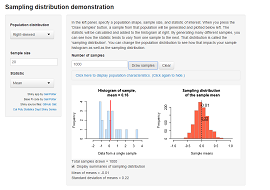Sampling Distributions of Various Statistics

This app allows the user to draw repeated samples from a specified population shape (normal, left-skewed, right-skewed, uniform, or bimodal). The user also specifies a statistic from the pull-down menu in the left panel. When a sample is generated by pressing the "Draw samples" button, a histogram of that sample is plotted in the graph at left, and the sample statistic is added to the sampling distribution histogram at right. The total number of samples is tracked at the bottom of the page, and the user may also elect to display the mean and standard deviation of the sampling distribution by checking the box. Above these two graphs, the user may also click to display the population curve and parameter of interest.

## Special Topics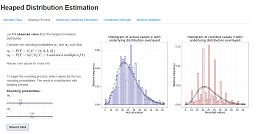Heaped Distribution Estimation

Data often exhibit a heaped distribution in situations when there are either rounding or recall issues. Then, heaping is observed in the distribution when there are unusual spikes at certain values. In this app, the focus is heaping present at multiples of 5. Two rounding behaviors are assumed and they are accounted for in the form of two rounding probabilities. The first rounding probability describes the tendency to round with smaller values, while the second rounding probability describes the tendency to round with larger values. Therefore, a mixture model is constructed with a specified distribution and the two rounding probabilities. Throughout the app, interpretations in popovers are provided for users to understand the different stages of the demonstration.

Users have the option to either simulate data or upload data to begin the app. There are five distributions for users to choose and the parameters can be adjusted. The proceeding tab describes the rounding process to users; the actual and rounded/heaped distributions are visually displayed for users to compare. With the heaped distribution, the goal for users is to estimate the actual distribution with maximum likelihood. After obtaining the estimates, confidence intervals can be produced either based on the inverse Fisher information matrix or bootstrapping. For users to validate the method, a simulation study can be performed in the last tab of the app. They can compare the means of the MLE distributions to the specified underlying parameters.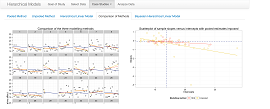Hierarchical Models

Hierarchical models are used when there is nesting of observational units in the data and variables are observed on multiple levels of the hierarchy. Failure to account for the hierarchy in the data may result in invalid conclusions. However, hierarchical models are not always needed for nested data as the intraclass correlation coefficient determines the requirement. This app focuses on illustrating the concept of hierarchical models by comparing the method to the two others at the extremes: the pooled and unpooled methods. Users are shown mathematically and visually how the hierarchical estimates are weighted averages and how they serve as a balance between the pooled and unpooled estimates; the two related ideas of shrinkage and borrowing strength are illustrated in this process.

Users have the capability to either use sample data sets or upload their own data to learn about hierarchical models through case studies. The three different scenarios for learning are varying-intercept, varying-intercept and varying-slope, and varying-intercept and varying-slope with level 2 predictor. In each scenario, users are first presented outputs and graphs of the pooled and unpooled method. Then they proceed to the hierarchical model and different concepts of this method are explained in compartments. Interpretations are included throughout the outputs for users to comprehend the ideas. Additionally, each scenario contains a comparison of the three modelling methods with visualizations. For those who are familiar with Bayesian methods, a tab is available to run a Bayesian hierarchical model. After grasping the concept of hierarchical models, users can analyze their own data with their own specified model.Population Genetics

Genetic drift, or the variation in the relative frequency of particular genotypes within a population, can lead to a higher prevalence or disappearance of certain alleles within a population. Genetic drift is often more visible within smaller populations when compared to larger populations. By randomly pairing observations to represent mating couples, this app simulates the genetic drift of an allele based on a starting population size and the fitness levels of each genotype. A second population may also be generated to make comparisons based on population size or fitness levels of certain genotypes.